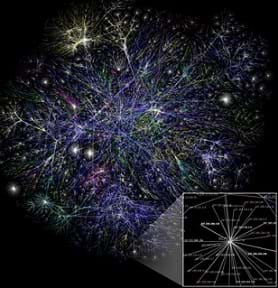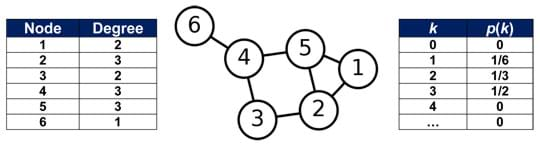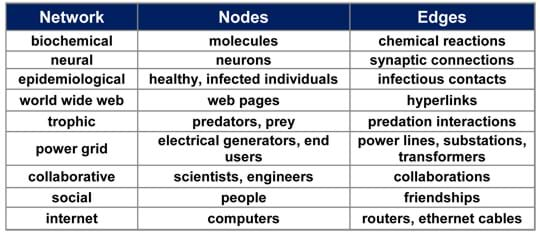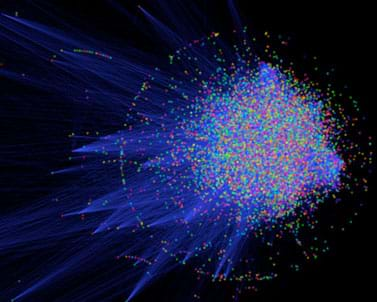# LessonSets-Nodes-Edges: Representing Complex Networks in Graph Theory

### Quick Look

Time Required: 45 minutes

Lesson Dependency: None

Subject Areas: Biology, Life Science, Problem Solving, Science and TechnologyNetworked systems, such as the internet, can be large and enormously complex. This poses a challenge to scientists and engineers who study such networks. Powerful mathematical concepts, such as graph theory, can be used to effectively analyze complex networks.copyrightCopyright © The Opte Project http://commons.wikimedia.org/wiki/File:Internet_map_1024.jpg

### Summary

Students learn about complex networks and how to represent them using graphs. They also learn that graph theory is a useful mathematical tool for studying complex networks in diverse applications of science and engineering, such as neural networks in the brain, biochemical reaction networks in cells, communication networks, such as the internet, and social networks. Topics covered include set theory, defining a graph, as well as defining the degree of a node and the degree distribution of a graph.

### Engineering Connection

Complex networks of interacting components are at the core of many problems of scientific and engineering interest, and this realization has caused the interdisciplinary study of networks to grow rapidly during the past decade. Neural engineers represent the connectivity of neurons in the human brain using graphs, whereas, electrical engineers use graphs to understand large and complex systems, such as the internet. The software engineers at Facebook use graphs to enable us to visualize social relationships, whereas, bioengineers study how an infectious disease, like the flu, might spread over a social network. Understanding the mathematics behind graph theory can help biomedical engineers and scientists to develop better cancer treatments and electrical engineers to design faster and more reliable communication networks among electronic devices.

### Learning Objectives

After this lesson, students should be able to:

• Provide examples of complex networks and formally represent them by graphs.
• Draw a visual representation of a graph and provide a formal description in terms of nodes and edges.
• Identify the degree of a node in a graph and calculate the degree distribution of the graph.

### Educational Standards Each TeachEngineering lesson or activity is correlated to one or more K-12 science, technology, engineering or math (STEM) educational standards. All 100,000+ K-12 STEM standards covered in TeachEngineering are collected, maintained and packaged by the Achievement Standards Network (ASN), a project of D2L (www.achievementstandards.org). In the ASN, standards are hierarchically structured: first by source; e.g., by state; within source by type; e.g., science or mathematics; within type by subtype, then by grade, etc.

###### Common Core State Standards - Math
• Describe events as subsets of a sample space (the set of outcomes) using characteristics (or categories) of the outcomes, or as unions, intersections, or complements of other events ("or," "and," "not"). (Grades 9 - 12) More Details

Do you agree with this alignment?

• Understand that a function from one set (called the domain) to another set (called the range) assigns to each element of the domain exactly one element of the range. If f is a function and x is an element of its domain, then f(x) denotes the output of f corresponding to the input x. The graph of f is the graph of the equation y = f(x). (Grades 9 - 12) More Details

Do you agree with this alignment?

• Use function notation, evaluate functions for inputs in their domains, and interpret statements that use function notation in terms of a context. (Grades 9 - 12) More Details

Do you agree with this alignment?

• Represent data with plots on the real number line (dot plots, histograms, and box plots). (Grades 9 - 12) More Details

Do you agree with this alignment?

###### International Technology and Engineering Educators Association - Technology
• Analyze the stability of a technological system and how it is influenced by all the components in the system, especially those in the feedback loop. (Grades 9 - 12) More Details

Do you agree with this alignment?

###### Florida - Science
• Explain how scientific knowledge and reasoning provide an empirically-based perspective to inform society's decision making. (Grades 9 - 12) More Details

Do you agree with this alignment?

###### National Council of Teachers of Mathematics - Math
• use representations to model and interpret physical, social, and mathematical phenomena (Grades Pre-K - 12) More Details

Do you agree with this alignment?

Suggest an alignment not listed above

### Introduction/Motivation

We all live in a connected world. Many, if not all, of us have cell phones capable of sending signals to nearby cellular towers, which can be bounced all over the world to potentially reach other people with phones. Likewise, we have access to computers, which can connect to other computers all over the world using the internet. While these networked systems of interconnected components are triumphs of modern engineering, nature has been producing large and complex networks for millions of years.

Can anyone think of other examples of complex networks? (Listen to student ideas. Use bullet points below for some answers and corresponding motivating discussion.)

• The human brain contains billions of neurons. Each neuron can transmit information to a large number (up to about 10,000) of other neurons through complex synaptic connections. Neural scientists are eager to understand how this complex network of interconnected neurons produces the vast complexity of human thoughts and consciousness.
• Molecules inside cells of living organisms, such as proteins, interact with each other through biochemical reactions to produce other molecules that regulate and control biological function. Networks of biochemical reactions are so complex that cell biologists struggle to decipher their structures and understand their functions (see Figure 2). Diseases, such as cancer, can occur when certain biochemical reactions fail to function properly. Understanding which reactions are responsible for the onset of a disease can lead to pharmacological therapies to repair malfunctioning biochemical reactions and thus help doctors treat the disease.
• Humans form social networks of various types: money flows through economic networks, diseases spread by infected individuals in contact with healthy individuals, information and ideas flow over friendship/acquaintance networks, such as Facebook, and beliefs and opinions are formed and modified based on conversations and discussions we have with trusted friends.
• Electricity is transmitted through elaborate and complex power grids from generators to entire countries, cities and individual end users. Building power grid networks that are resilient to intense weather phenomena and robust to increasing electricity demands (for example, during summer months when air conditioning use is high) is of great importance. A continuous and stable supply of this important commodity enhances our standard of living and enables us to pursue our everyday activities with no major interruptions.

Network scientists and engineers spend a great deal of time studying complex networks, such as these. The size and complexity of these systems means that a network scientist or engineer cannot rely only on her intuition when she tries to design, analyze and understand their functional properties. Important mathematical concepts, such as graph theory, are used extensively by scientists and engineers to develop a common mathematical framework for modeling complex networks. Using this framework, they can effectively communicate with each other and develop techniques and software for studying the properties and design principles of complex networks using powerful computers.

Next, we introduce the concept of a graph, which is at the foundation of graph theory, and discuss how this mathematical construct is used to represent complex networks. (Continue on to present to students the content on set theory, graphs and degree distribution covered in the Background section, and the associated assessments.) Following the lesson, encourage students to conduct the fun associated activity Start Networking! where they create and analyze an example complex network by interacting with their peers in the classroom and documenting the interactions.

### Lesson Background and Concepts for Teachers

A Brief Overview of Set Theory

It is necessary for students to be familiar with the mathematical concept of sets. It is not however necessary for students to understand all details of set theory. For additional information, see "Set (mathematics)" at Wikipedia.

A set is a collection of well-defined distinct objects. Mathematically, a set is represented as a list of objects contained inside curly braces, {}, and separated by commas. So, the set S = {1, 2, 3} contains the first three natural numbers, 1, 2 and 3, as its members. We say that 1 is an element of the set S, and we write this as 1 ∈ S. Any given object is either a member of a given set or it is not. For example, 4 is not an element of the set S, and we write this as 4 ∉ S. The order of the items in a set does not matter, so S = {1, 2, 3} = {3, 1, 2} = {3, 2, 1}.

The objects in a set need not be numbers. For example, students in a class can form a set C = {John, David, Anne, Debbie}. Note that the objects in a set must be distinct. If the class has two students named John, then the set should include them both by identifying them as distinct individuals. For example, C = {John Smith, John Doe, David, Anne, Debbie} is a set but C = {John, John, David, Anne, Debbie} is not.

Importantly, a set is considered an object in its own right, and thus one can create a set of sets. For example, if the history class is given by H = {John, David} and the math class is given by M = {David, Laura}, then we can also define the set of sets S = {H, M} = {{John, David}, {David, Laura}}. It is crucial that students understand this last point before moving to the topic of graphs, since the set of edges in a graph is a set of sets.

Before moving to the next topic, verify that students understand the basics of set theory by conducting Assessment 1: Sets, as described in the Assessment section.

Defining a Graph

Much confusion could arise due to the word "graph" most commonly being used in middle school mathematics to refer to a plot of a function. In graph theory, a graph has nothing to do with plotting functions. Instead, a graph is a mathematical structure that models pairwise relations between objects in a certain set. The visual representation of an example graph helps to immediately clarify this distinction. For example, consider the graph in Figure 1.Figure 1. A visual representation of a graph. Labeled circles represent nodes. Lines that connect two nodes represent edges. The left table indicates the degree of each node of the graph. The right table indicates the degree distribution for the graph.copyrightCopyright © (graph) User:AzaToth {PD} http://commons.wikimedia.org/wiki/File:6n-graf.svg

Figure 1 clearly demonstrates that in graph theory a graph is something different than the standard plot of a function. A graph is mathematically defined as the pair G = (N, E), where N is a set of nodes and E is a set of edges connecting the nodes. In Figure 1, the set of nodes is given as N = {1, 2, 3, 4, 5, 6}. It is standard to represent the nodes as labeled circles. The location of these circles in the visual representation of the graph is arbitrary; all that matters is that the edges connect the correct nodes together. An edge is defined as a set with two elements from the set N. In Figure 1, the lines between nodes represent the edges of the graph. The line between node 1 and node 2 means that {1, 2} is an edge of graph G, and we write this as {1, 2} ∈ E. We can specify the set of edges as: E = {{1,2}, {1,5}, {2,5}, {3,2}, {3,4}, {4,5}, {4,6}}, which is clearly a set of sets.

Before moving to the next topic, verify that students understand the mathematical and visual representation of graphs by conducting Assessment 2: Graphs, as described in the Assessment section.Table 1. Example real-world complex networks, with their nodes and edges identified.

Once students complete Assessment 2, ask them to identify some examples of real-world, complex networks and represent them as graphs. Table 1 provides many examples of how graphs could be used to represent real, complex networks. For example, protein networks in cells can be represented by a graph, such as the one depicted in Figure 2, with the nodes representing proteins and the edges connecting interacting proteins.Figure 2. A graph helps to visualize protein-protein interactions in human cells. Each node represents a protein and each blue line between them represents an interaction.copyrightCopyright © Keiono http://commons.wikimedia.org/wiki/File:Human_interactome.jpg

Another visual example is shown in the "internet map" image at the beginning of the lesson write-up—a graphical representation of a portion of the internet on a day in 2005. Lines are drawn between nodes, each representing connected IP addresses (for devices such as computers or printers) in the internet.

Degree of a Node and Degree Distribution of a Graph

Now that we have defined graphs, we can try to understand more about them by calculating some useful mathematical quantities. An important mathematical quantity of great interest to network scientists is the degree of a node. This is simply the number of edges that connect to the node. The left table in Figure 1 gives the degree of each node of the graph.

The degree of a node is a measure of its importance. Often, the larger the degree of the node, the more important the node seems to be. For example, Google is an important node in the World Wide Web, since a great number of web pages are connected to it at a given time (via hyperlinks). If this node were compromised (for example, by malicious hackers or computer viruses) it would interrupt the traffic on the web and be very disruptive in our lives. The disruption would be much more severe than if your Facebook profile experienced problems, since the number of people visiting a profile is miniscule compared to the number of people visiting Google. As a consequence, engineers around the world spend substantial effort to protect the integrity of the World Wide Web, and a large portion of that effort is focused on protecting important nodes, such as Google. Graph theory is a mathematical tool that can be used to identify important nodes in a complex network by computing, for example, their degrees in the graph representing the network.

Another important mathematical quantity of great interest to network scientists is the degree distribution of a graph. If we let k be a number representing the degree of a node (this number can only take integer values 0, 1, 2, etc.), then the degree distribution of a graph is a function p(k) that tells us what fraction of the nodes in the graph have degree k. For example, the right table in Figure 1 shows the degree distribution for the graph.

Interestingly, many large networks have degree distributions that are highly right-skewed, meaning that a large majority of nodes have low degree, while a small number of nodes have high degree. High degree nodes represent "hubs'' that control many properties of the network as a whole and are important points of study by network scientists and engineers.

To conclude, verify that students understand the concepts of degree of a node and degree distribution of a graph by conducting Assessment 3: Degree Distribution, as described in the Assessment section:

### Associated Activities

• Start Networking! - Students create and analyze an example complex network by interacting with their peers in the classroom and documenting the interactions. They represent the data as a graph and calculate the degree of each node and the degree distribution of the graph. Then they analyze the graph and these quantities to see what it tells them about their social network.

### Lesson Closure

In this lesson, we have introduced complex networks and discussed their importance in many different science and engineering disciplines. We also discussed how to mathematically represent a complex network by a graph. A graph captures the information about which individuals (nodes) in the complex network interact with one another (determined by the edges).

We have not yet discussed how individuals interact with each another, since this usually depends on the given application. In the next lesson, we will discuss how a process propagates on a complex network as a consequence of how individuals interact with each other. As an example, we will study how a contagious disease, such as the flu, propagates on a social network of interacting students in a school.

### Vocabulary/Definitions

complex network: A set of individuals (students, neurons, molecules, computers, web pages) that interact with each other in a certain fashion.

degree distribution: A function telling what fraction of the nodes in a graph has a given degree.

degree of a node: The number of edges connecting to the node.

graph: A mathematical structure that models pairwise relations between objects in a certain set. Composed of a set of vertices and a set of edges that connect the vertices. Graphs are used as visual representations of complex networks.

set: A grouping of well-defined, distinct objects.

### Assessment

Assessment 1: Sets (before moving to graphs)

To test whether students understand the concept of a set, quiz them on which of the following objects are sets and which are not. Require that they explain why.

• S = {-1, 0, 1} (Answer: This is a set.)
• S = {345345, 4, -34.535, 0} (Answer: This is a set.)
• S = {1, 2, 3, 4, 3, 2, 1} (Answer: This is not a set, since the objects 1, 2, 3 appear more than once and are not distinct.)
• S = {{}, {1, 2}, {John, 2, \$}} (Answer: This is a set of sets, and thus it is a set; {} is the empty set [it has no elements], {1, 2} is a set, {John, 2, \$} is also a set since all objects are distinct.)

Assessment 2: Graphs (before moving to examples)

Verify that students understand the mathematical and visual representation of graphs. For a graph drawn on the classroom board, have students correctly define the set N of its nodes as well as the set E of its edges. Conversely, given a set of nodes N and a set of edges E, expect students to be able to draw the graph with labeled circles (nodes) connected by lines (edges). Refer to Figures 1 and 2 for graph examples. Next: Ask students to identify real-world, complex networks and represent them as graphs (see Table 1 examples.)

Assessment 3: Degree Distribution (at lesson end)

Draw a graph on the classroom board. Have students list the nodes and their corresponding degrees. Then, have them plot the degree distribution p(k) as a function of k, or ask them to provide its values in a table.

### Subscribe

Get the inside scoop on all things TeachEngineering such as new site features, curriculum updates, video releases, and more by signing up for our newsletter!
PS: We do not share personal information or emails with anyone.

### More Curriculum Like This

High School LessonProcesses on Complex Networks

Building on their understanding of graphs, students are introduced to random processes on networks. They walk through an illustrative example to see how a random process can be used to represent the spread of an infectious disease, such as the flu, on a social network of students.

High School LessonMaking the Connection

Students learn and apply concepts and methods of graph theory to analyze data for different relationships such as friendships and physical proximity. They are asked about relationships between people and how those relationships can be illustrated.

High School LessonGraph Theory in Drama

Students use graph theory to create social graphs for their own social networks and apply what learn to create a graph representing the social dynamics found in a dramatic text.

High School ActivityStudents analyze their social networks using graph theory. They gather data on their own social relationships, either from Facebook interactions or the interactions they have throughout the course of a day, recording it in Microsoft Excel and using Cytoscape (a free, downloadable application) to gen...

### Contributors

Garrett Jenkinson and John Goutsias, The Johns Hopkins University, Baltimore, MD; Debbie Jenkinson and Susan Frennesson, The Pine School, Stuart, FL

### Supporting Program

Complex Systems Science Laboratory, Whitaker Biomedical Engineering Institute, The Johns Hopkins University

### Acknowledgements

The generous support of the National Science Foundation, Directorate for Computer and Information Science and Engineering (CISE), Division of Computing and Communication Foundations (CCF), is gratefully acknowledged.